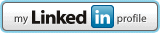Under Construction

# Math 1140 (10-week course)

## Course Description

An accelerated course in college algebra in 10 weeks (followed by trigonometry in 6 weeks). Algebra topics covered include linear equations, rational functions, radicals, quadratic equations, inequalities, determinants, progressions, theory of equations, permutations, combinations, and the binomial theorem.

Syllabus      Course Calendar

## Semesters

 Fall 2012 Fall 2013 Fall 2014

## General Materials

 A Brief History of Algebra Theorems for Factoring Polynomials Asymptotes and Analyzing Graphs of Rational Functions All About Asymptotes About Conics Drawing a Parabola from Definition Why Satellites are Parabola-Shaped Why Euler's Identity? Word Problem Formulas (Financial Models and Growth/Decay Models) Graphing Grid (5x5) Graphing Grid (10x10)

## Exams

 Fall 2012 Exam 5 Fall 2014 Exam 1Solution Exam 2Solution Exam 3Solution Exam 4Solution Exam 5Solution

## Quizzes

 Fall 2012 Quiz 1 Solution Quiz 2 Solution Quiz 4 Quiz 5 Quiz 6 Fall 2014 Quiz 1 Solution Quiz 2 Solution Quiz 3 Quiz 4 Solution Quiz 5 Solution

## Worksheets

 Inequalities Worksheet Solution

Contact Information
email: bcuchta@fairmontstate.edu or bcuchta@gmail.com 423 Engineering Technology Building, Fairmont State University